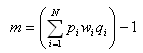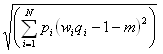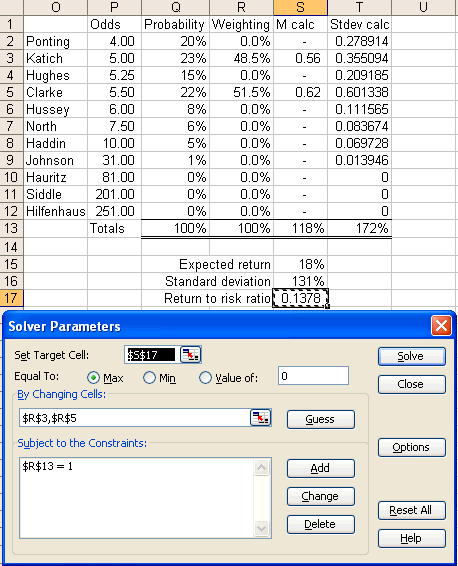# Betting Strategies – Part 2 – Maximizing Expected Return to Risk Ratio

In the first part of this two post series, I discussed the betting strategy of maximizing expected return. The downside of this strategy is it takes no account of risk. If you haven’t read the previous post I recommend you do so before continuing.

This post outlines how to maximize your expected return to risk ratio. I highlight the use of the word ‘expected’ because it involves deriving your own probabilities for each of the expected outcomes.

Let’s look again at the ‘high bat’ for Australia’s first innings in the second Ashes test in 2009. As before, consider each outcome in turn and determine your own probability that each option will occur. Then run the calculation (ODDS x PROBABILITY) – 1 to determine your expected return for each pick.

 Batsman Bookmaker odds Your probability Expected return Ricky Ponting 4.00 20% -20% Simon Katich 5.00 23% 15% Phillip Hughes 5.25 15% -21% Michael Clarke 5.50 22% 21% Michael Hussey 8.00 8% -52 Marcus North 7.50 6% -55% Brad Haddin 10.00 5% -50% Mitchell Johnson 31.00 1% -69% Nathan Hauritz 81.00 0% -100% Peter Siddle 201.00 0% -100% Ben Hilfenhaus 251.00 0% -100% Total probability 100%

If you placed an equal bet on each player, your expected return would equal the average of the individual returns (-20% + 15% + …) / 11. Based on the probabilities above this equals -48%. If you wanted to maximize expected return you would bet on Clarke and no one else to provide an expected return of 21%. The problem with this strategy, however, is that it involves a considerable amount of risk. Risk, in this case, can be measured by the standard deviation of returns. Your standard deviation would equal 228%, which is huge! Your reward to risk ratio would be 21% / 228% = 0.09.

You may be able to achieve a higher reward to risk ratio by betting on more than one cricketer. As I have alluded to earlier this equates to maximizing your expected return to standard deviation ratio.

To be able to determine your best strategy, you will first need to know how to calculate expected return and standard deviation for a combination of bets.

Let Pi denote the probability that event i occurs. If there are eleven possible outcomes, as in the case here, you have

P1 + P2 + P3 + P4 + P5 + P6 + P7 + P8 + P9 + P10 + P11 = 1

Where each Pi is between 0 and 1.

More generally, we can represent this asLet Wi denote the proportion of your total bets for this event that you place on a particular outcome. If you place a bet on only one player this equals 1 for that player and 0 for everyone else. The sum of your bet weightings will also equal 1.

As an example if you made equal bets on Clarke and Katich you would bet

W1 + W2 + W3 + W4 + W5 + W6 + W7 + W8 + W9 + W10 + W11 = 1

0 + 0.5 + 0 + 0.5 + 0 + 0 + 0 + 0 + 0 + 0 + 0 = 1

Again, we can express this more generallyNow, let Qi denote the bookmaker’s odds for each bet.

So for Ponting, which we will set as i = 1
P1 = 20%
W1 = ?
Q1 = 4.00

We don’t know what the actual weight is for each player yet, hence we don’t know W1, or any of the other weights.

To calculate our expected return if we bet a weighing Wi on each player, we use the formulaSo if we bet half our money on Katich and the other half on Clarke we would have

Katich
W2 = 0.50
P2 = 0.23
Q2 = 5.00

Clarke
W4 = 0.50
P4 = 22%
Q4 = 5.50

All of the other figures would equal zero for the other players because their weightings are zero.

Our expected return would equal

m = [(0.23)(0.50)(5.00) + (0.22)(0.50)(5.50)] – 1 = 18%

To calculate our standard deviation we use the formulaThe problem with this formula is it involves a large number of variables, because even if you don’t bet on a particular outcome, it still has an impact on the calculation. The best way to calculate your standard deviation, and in turn your optimal strategy, is to use spreadsheet software like Microsoft Excel.To calculate expected return, column E calculates probability x odds x weighting for each outcome. Cell E13 sums these figures and cell E15 calculates the expected return, m for a particular series of bets, by subtracting 1 from E13. Refer to the relevant formula above for clarification.

To calculate standard deviation, column F calculates probability x (odds x weighting – 1 – m)^2 for each outcome. m is simply the value in E15. Cell F13 sums these figures and cell E16 takes the square root to provide the standard deviation.

In the example above a bet on Clarke would have an expected return of 21% with a standard deviation of 228%. As I have mentioned before, this provides a reward to risk ratio of 21% / 228% = 0.09.

To calculate the combination of bets that provides the best reward to risk ratio, you need to use the Excel Solver feature. To make this feature available, select Tools -> Add-Ins in the menu bar. Select the Solver Add-In and click on OK. To use the solver, select Tools -> Solver.The solver feature attempts to set a target cell equal to a certain value by manipulating other selected cells. For this example I have used the solver to find the maximum possible value for the reward to risk ratio by manipulating the betting weights. I have set it to only manipulate the weights for Clarke and Katich because they are the only ones that provide a positive expected return. Also note that I have set a constraint so that the sum of the weights equals 1.By placing 48.5% of your bet on Katich and 51.5% on Clarke you have an expected return of 18% and a standard deviation of 131%. This provides a return to risk ratio of 0.14, which is a substantial improvement on 0.09 if you only placed a bet on Clarke. We have reduced our risk drastically and have only dropped our expected return from 21% down to 18%. This is a marked improvement on the maximized expected return strategy.

As you can see, this strategy requires that you be familiar with spreadsheet software. I recommend you setup a template spreadsheet so you can run calculations on various events with ease. If you have any questions about how to implement this strategy in Excel, feel free to ask.

I must reiterate that this strategy is heavily dependent on your inputted probabilities of success for each outcome. If you get these right then this strategy will pay handsome dividends.